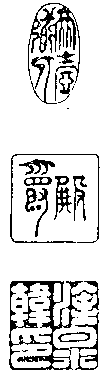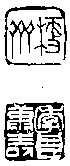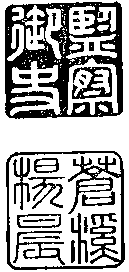# 天台山方外志• 韓將軍序

• 始豐張序

• 翰林院楊序

• 王邑侯序

• 虞司勳序

• 屠儀部序

• 翰林院顧序

• 幽溪燈序

• 第一卷

• 山名考第一

• 山源考第二

• 山體考第三

• 第二卷

• 形勝考第四

• 山（五十三處）

• 第三卷

• 峰（三十三處）

• 巖（二十九處）

• 嶺（四十處）

• 洞（八處）

• 石（十處）

• 溪（二十五處）

• 潭（二十處）

• 湖（八處）

• 塘（三處）

• 井（九處）

• 泉（十處）

• 池（三處）

• 第四卷

• 山寺考第五

• 寺（六十二所）

• 院（九所）

• 庵（十四所）

• 蓮社（一所）

• 道場（二所）

• 堂（二所）

• 觀（五所）

• 宮（五所）

• 道院（十二所）

• 道庵（三所）

• 第五卷

• 聖僧考第六

• 隱（二處）

• 顯（二十一人）

• 補遺（七人）

• 第六卷

• 祖師考第七（一十七人）

• 第七卷

• 台教考第八

• 教觀大綱

• 教門（十[A7]八條）

• 觀門（九條）

• 清規（二條）

• 教觀書目（共六十三人書三百部）

• 石室藏書（儒部四十一種仙部十九種志部十一種）

• 第八卷

• 高僧考第九

• 教宗（五十八人）

• 禪宗（五十七人）

• 蓮宗（十四人）

• 律宗（人）

• 第九卷

• 神仙考第十

• 神仙（三十六人）

• 道士（三十七人）

• 第十卷

• 隱士考第十一

• 隱士（五十人）

• 僑寓（二十三人）

• 神明考第十二

• 護伽藍神（二人）

• 龍神（十人）

• 土谷神祗（十七人）

• 第十一卷

• 金湯考第十三（七十七人）

• 第十二卷

• 盛典考第十四（二十五事）

• 靈異考第十五

• 釋（三十四事）

• 道（三十事）

• 第十三卷

• 塔廟考第十六（三十九處）

• 佛塔（一十三處）

• 祖塔（二十六處）

• 墳墓

• 古蹟考第十七（六十七處）

• 碑刻考第十八

• 碑（六十七處）

• 手書（四種）

• 巖鐫（十四人）

• 異產考第十九

• 食（十六種）

• 木（五種）

• 杖（三種）

• 花（五種）

• 草（四種）

• 獸（十三種）

• 禽（十四種）

• 藥（七十九種）

• 石（三種）

• 第十四卷

• 文章考第二十

• 敕（十五首）

• 第十五卷

• 書（二十二首）

• 第十六卷

• 書（三十三首）

• 第十七卷

• 書（二十九首）

• 第十八卷

• 疏（十二首）

• 第十九卷

• 序（三十一首）

• 第二十卷

• 記（十六首）

• 第二十一卷

• 記（十六首）

• 第二十二卷

• 記（首）

• 第二十三卷

• 碑（六首）

• 第二十四卷

• 塔銘（五首）

• 行狀（一首）

• 傳（一首）

• 第二十五卷

• 贊（十三首）

• 第二十六卷

• 賦（四首）

• 第二十七卷

• 詩（賦景）

• 天台山（四十二首）

• 第二十八卷

• 國清寺（二十八首）

• 赤城（二十四首）

• 佛隴諸寺（九首）

• 華頂（十三首）

• 石梁橋（三十三首）

• 萬年寺（一十二首）

• 玉霄峰（三首）

• 桐柏宮（三十八首）

• 瓊臺（二十三首）

• 第二十九卷

• 桃源洞（三十三首）

• 護國寺（七首）

• 寒巖（十六首）

• 明巖（六首）

• 雜詩（九首）

• 曹文晦八景（八首）

• 第三十卷

• 寄贈（六十首）

• 佛隴曦跋

(刻板餘貲印訂三佰部流通)

# 校注

 「牧」張智等編輯《中國佛寺志叢刊81天台山方外志》作「彼」（揚州廣陵書社2006此版簡稱「廣陵本《天台山方外志》」應用於後不再贅述  「朿」廣陵本《天台山方外志》作「束」  「英」廣陵本《天台山方外志》作「莫」  「雪居」底本 p. 160p. 198 等皆作「雲居」  「摸」廣陵本《天台山方外志》作「摹」  「愰」廣陵本《天台山方外志》作「幌」  編按底本僅存目無內文  《山記》即《南嶽記》  天冠菩薩住處另有他說《釋氏通鑑》卷第十二「福州支提山乃天冠菩薩道場」(CBETA, X76, no. 1516, p. 132, a6-7)  編按底本「得」字疑為「待」字
[A1] 市【CB】巿【志彙】
[A2] 幔【CB】慢【志彙】
[A3] 干【CB】千【志彙】
[A4] 冪【CB】[幕-卄+(ㄇ@(企-止))]【志彙】
[A5] 已【CB】巳【志彙】
[A6] 瘞【CB】[療-(日/小)+土]【志彙】
[A7] 八【CB】入【志彙】